# Writing a Proof: Substance, then Style

#### (A new question of the week)

A question from last month provides an opportunity to show how to develop an algebraic proof of a combinatorial identity involving factorials. We’ll be looking over Doctor Rick’s shoulder as he guides a student through the maze. I’ll also add in a previously published version of the same proof for comparison, and a combinatorial proof of the same fact.

## A combinatorial identity

Here is the question, from Frankie:

Hi. I’m having some problems answering this question.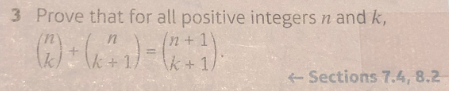I’ve included a picture of my workings so far but I’ve hit a wall and can’t think how to carry my work on to finish the proof. Any hints or tricks for this question would be appreciated.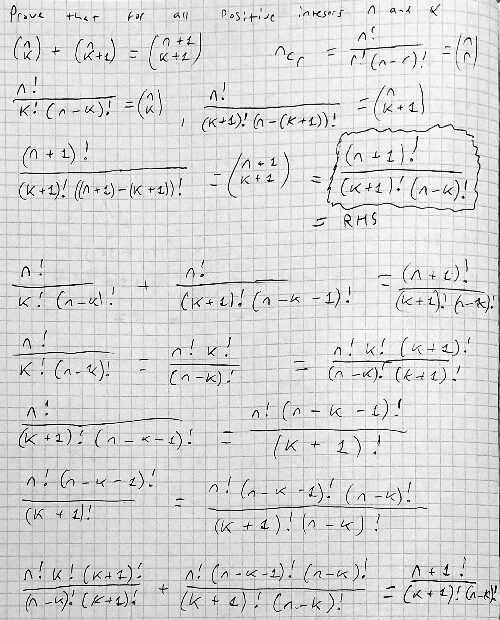Also I will include a picture of a possible way to proceed, however I’m unsure if it is numerically sound.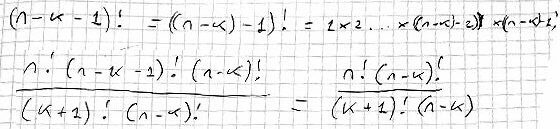The top third of the first page restates the problem in terms of factorials, using the fact that $${n\choose k}=\frac{n!}{k!(n-k)!}$$ Then each term has been rewritten, with some invalid moves.

Let’s first think about what the identity says: $${n\choose k} + {n\choose {k+1}} = {{n+1}\choose{k+1}}$$

This says that the number of ways to choose k + 1 out of n + 1 items is the same as the number of ways to choose k out of n items, plus the number of ways to choose k + 1 out of n items. We can see examples of this in Pascal’s Triangle: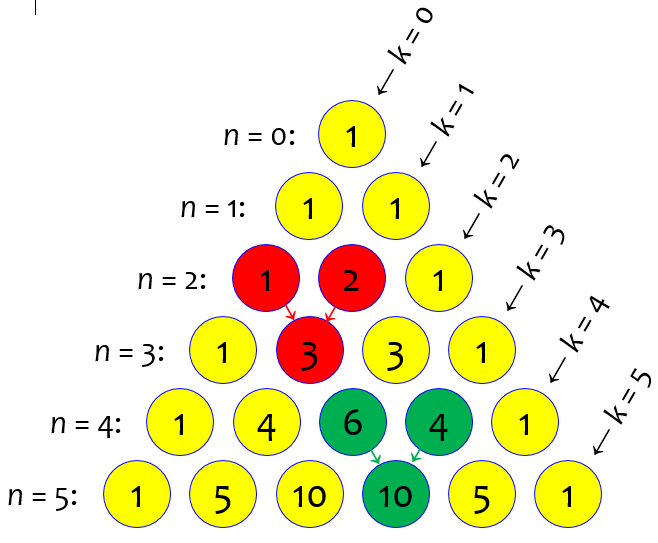As illustrated in red and in green, the identity says that the sum of two adjacent numbers in the nth row is the number between them in the (n+1)st row. This is the quick way to create the triangle.

The green case, for example, which I’ll be demonstrating more fully later, shows that $${4\choose 2} + {4\choose 3} = {5\choose 3}$$

Hi, Frankie.

You started well, expanding each combination in terms of factorials, but after that I can see that you are floundering, not finding a way to make any progress toward connecting the two sides.

The secret ingredient you’re searching for is this:

(n+1)! = (n+1) n!

which could be taken as a recursive definition of the factorial, along with a starting point, 1! = 1 (or 0! = 1).

This may be all the help you need. Give it another try, and let me know if you get stuck again. (I’d like to know if you succeed, also!)

This identity simply reflects the fact that $$(n+1)! = (n+1)(n)(n-1)…(3)(2)(1) = (n+1)\left((n)(n-1)…(3)(2)(1)\right) = (n+1)n!$$ For example, $$5! = 5\cdot 4\cdot 3\cdot 2\cdot 1 = 5\cdot (4\cdot 3\cdot 2\cdot 1) = 5\cdot 4!$$. It is very useful in simplifying expressions using factorials.

Frankie wanted to confirm a very relevant example:

Could you write (n-k)! = (n-k) (n-k-1)! ?

Doctor Rick replied,

Yes … now, do you see how to use this idea to pull out common factors in the denominators, etc.?

Frankie wanted a little more confirmation:

I think I have the main idea; before I continue, though, could you see if the picture I’ll include is correct?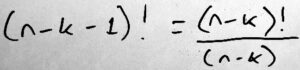Doctor Rick confirmed its truth while recommending against bringing division into the work, if only because division makes things a little more complicated:

Yes, that’s correct, although I am more inclined to work with multiplication and factoring rather than division, as in

(n–k)! = (n–k) (n–k–1)!

Frankie got back to work, and asked for a quick check:

I’m a bit stuck again as I feel like whatever I do I’m ending up with more terms on the fraction. I’ve simplified the fraction for the picture; if it is right could you perhaps give me a hint on where to go from here?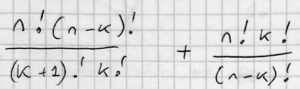Each term has an unclear provenance. It’s time to dig into details. Doctor Rick answered,

I don’t see where this could have come from – how you could get a product of two factorials in the numerator, or only one in the denominator.

We started with this as what we want to prove (it’s what I got, and I believe you got it too):

    n!             n!             (n+1)!
--------- + --------------- =? ------------
k! (n-k)!   (k+1)! (n-k-1)!   (k+1)! (n-k)!

Now, on the left side, we want to find the greatest common factor, as an aid in choosing the least common denominator. For this purpose, I would write (k+1)! as (k+1) k!, and (n-k)! as (n-k) (n-k-1)!, so that we can see the common factor k! (n-k-1)!.

This gives us a specific goal. We have the tool that lets us express a factorial in terms of a smaller one; now we have a reason to use it, namely to break down each factorial into smaller ones that will be shared by the terms.

Frankie took that starting point and gave it a new try:

Okay, I restarted and here is what I’ve got so far: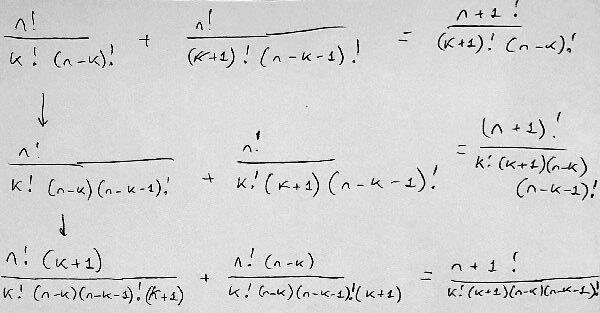Frankie is working on both sides of the identity at once (which we’ll be commenting on later), applying our key fact and then forming a common denominator. All the steps are valid, so we’re making progress. Then, ten minutes later:

Here is what I’ve got to now. I think I’m pretty close to the answer now?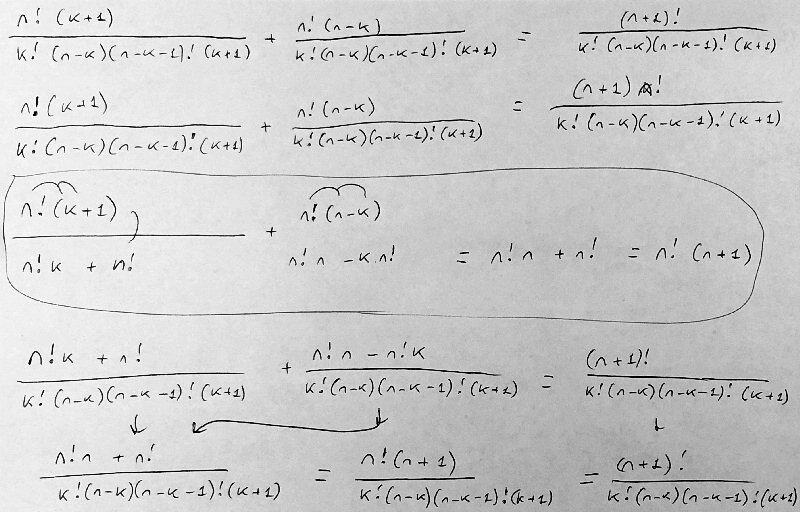Frankie has distributed and combined terms, and does indeed seem close. Doctor Rick replied with a suggestion for making the work clearer (and therefore safer):

Your work is somewhat hard to follow because (I think) you keep writing both the left and right sides of what we are trying to prove, though you haven’t yet shown that they were equal. When you do that, it’s hard to be sure which expressions you’re saying you know to be equal.

The usual practice, in presenting a proof like this, is to start with the expression on one side, and rewrite it step by step until what you’ve got is the right side of what you were to prove. I understand that you aren’t at the stage of writing up your proof yet, and while you’re exploring, it is helpful to keep in view the target you’re aiming at — the right side. There are two things I might do to help me keep things straight.

1. I might write those equal signs as you did, but put a question mark above the equal sign if it’s one I want to prove rather than one I know to be valid. (In my previous message I wrote =? with that idea.)
2. I might write the identity to be proved at the top of the page, then draw a vertical line down the page where the equal sign is, to keep the two sides separate until they turn out to be the same.

Anyway, back to the work you show. I can’t make sense of the work that you circled, but ignoring that, your work seems to be correct. Having gotten a common denominator, you needed to add the numerators, which you did, but it could have been done a bit more simply like this:

n!(k+1) + n!(n-k) = n!(k+1+n-k)

= n!(n+1)

= (n+1)!

We’ve previously discussed proofs of a trigonometric identity, and alternate ways to present them; some of the ideas here are similar. The work at the end replaces Frankie’s distribution with it opposite, factoring. Both are valid.

Frankie took the line-down-the-middle approach, but still worked on both sides:

Hi I rewrote it out neatly here with anything to the left of the red line being my work so to speak and to the right is the RHS (i.e. what the LHS needs to look like). If my methodology is correct I will rewrite the proof to be more presentable; however, for now I just want to see if I’ve got it right.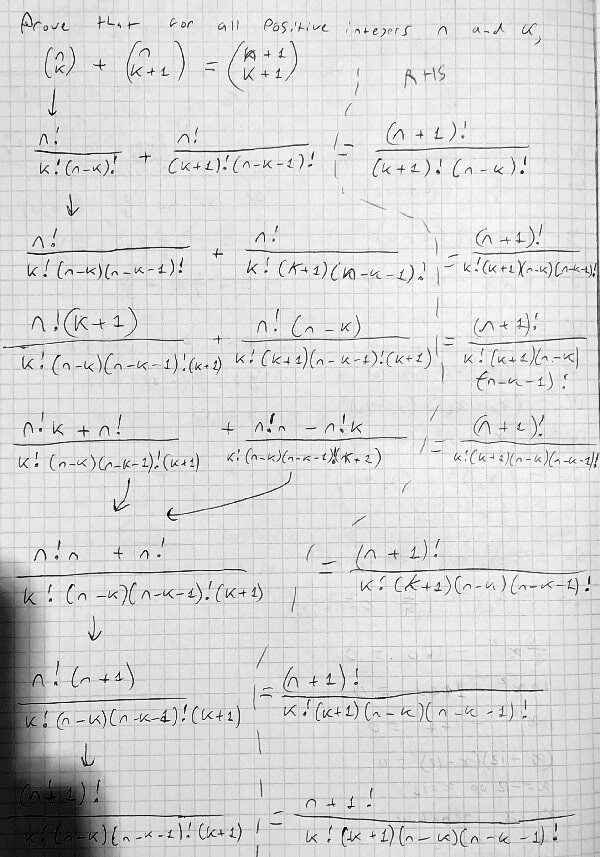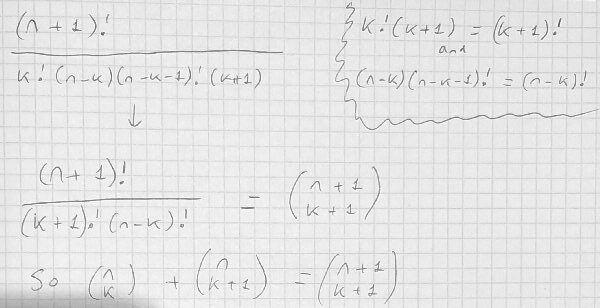Doctor Rick acknowledged that the work is (mostly) correct, with a couple comments:

You miswrote the fourth line, I now notice: the denominator of the second fraction has two factors of (k+1), one of them was supposed to be (n-k). But I know you were thinking correctly, and just wrote something different, because the next line is correct.

On the second page you correctly wrap up the proof, essentially reading up the right column on the first page. That’s how I think of this sort of proof: as a U in which the final proof starts at the upper left, goes down around the bottom of the U and back up to the top right.

Looks good!

The U idea is also discussed in the trig identity post. Below, we’ll be seeing what amounts to a final cleaned-up version of this proof.

## Another look at the algebraic proof

In working this into a post, I was going to just type up the final version for clarity, but then discovered that we have a similar algebraic proof of the same identity in Ask Dr. Math. It may be enlightening to compare it.

Here is the question, from 1998:

Pascal's Triangle and Combinations

In Pascal's Triangle, there is a pattern where any given number from the triangle is the sum of two numbers in the row before it that are closest to the number. So in the fourth row down from the top, in the third column, the number is 6. The two numbers closest to it in the previous row are 3 and 3. So 3 + 3 = 6.

Each number from the triangle can represent a combinations problem. In this case, the 6 is equal to 4 C 2. The threes are equal to 3 C 1 and 3 C 2.

Why is 4 C 2 equal to the sum of 3 C 1 and 3 C 2?  I realize that 3 plus 3 is 6, but I need a detailed explanation that shows how 3 C 1 + 3 C 2 equals 4 C 2. I have come up with a formula:

x combinations taken y at a time = (x-1) C (y-1) + (x-1) C y

However, this hasn't helped much in my understanding of the relation. Please give me any information you have on this relation of a number in Pascal's Triangle and the two numbers closest to it in the previous row, in terms of how they are represented in combinations.

Jesse had been shown this pattern in Pascal’s Triangle without proof, and has been able to express it as a formula, using the notation $$_n\text{C}_k = {n\choose k}$$, but now wants to understand it. Bravo!

Doctor Pat answered, giving a proof in terms of factorials as above:

Jesse,

I'll try to do all this in a way that you can see it develop.  Here goes:

I hope the value of n C r  = n! / ( r! (n-r)!) is already known to you. I will use it to show why the relation you observe is true. First, though, we'll do an example to make a believer of you.

Let's look at 8 C 3, 8 C 4 and note that the number below and between them is 9 C 4. Can you see that it will always be true that if the first two numbers are n C r and n C r+1, the number between and below will be n+1 C r+1?

Jesse has already seen numerical examples, but here the goal will be to prove it for this numerical case, as a template for the proof. I like this approach to formulating a proof in an unfamiliar area, by doing a “dry run” before diving in to formal details. In particular, by using specific numbers, we can more easily see what is happening with the factorials.

Back to our example: 8!/(8-3)!*3! ends up looking like this:

8*7*6
-------
3*2*1

Note that there will be the same number of values on the top and bottom, and with n C t, there are t numbers on top and bottom. So 8 C 3 + 8 C 4 looks like:

8*7*6    8*7*6*5
------- + -------
3*2*1    4*3*2*1

From here we have to do some simple fraction work. The two denominators are alike except for the first term of the second (4).  If we multiply the left fraction top and bottom by four (multiply by 4/4 = 1) we get:

4*8*7*6   8*7*6*5    4*(8*7*6) + (8*7*6)*5
------- + ------- =  ---------------------
4*3*2*1   4*3*2*1          4*3*2*1

Notice that on the right side I used parentheses to make a grouping show up.

If we factor the (8*7*6) we end up with 9 (8*7*6) which is the numerator of 9 C 4. Thus the whole thing is equal to 9 C 4.

So the work looks like this, finishing it up: $${8\choose 3}+{8\choose 4}=\frac{8\cdot 7\cdot 6}{3\cdot 2\cdot 1}+\frac{8\cdot 7\cdot 6\cdot 5}{4\cdot 3\cdot 2\cdot 1}=\frac{4\cdot (8\cdot 7\cdot 6)}{4\cdot 3\cdot 2\cdot 1}+\frac{(8\cdot 7\cdot 6)\cdot 5}{4\cdot 3\cdot 2\cdot 1}=\frac{9\cdot (8\cdot 7\cdot 6)}{4\cdot 3\cdot 2\cdot 1}={9\choose 4}$$

Now rewriting this with (n C r) + (n C r+1) to show it is equal to n+1 C r+1 looks full of variables, but remember the variables just stand for the numbers we used above:

(n C r) + (n C r+1)

n*(n-1)*...*(n-r+1)   n*(n-1)*...*(n-r+1)*(n-r)
= ------------------- + --------------------------
r*(r-1)*...*1        (r+1)*r*(r-1)*...*1

Now we multiply the left term by (r+1) and use square brackets for the special grouping to factor:

(r+1)*[n*(n-1)*...*(n-r+1)]   [n*(n-1)*...*(n-r+1)](n-r)
= --------------------------- + ---------------------------
(r+1)*r*(r-1)*...*1           (r+1)*r*(r-1)*...*1

With the square brackets, we see that the numerator is:

[(r+1)+(n-r)]*[n*(n-1)*...(n-r+1)] = [n+1]*[n*(n-1)*...(n-r+1)]

which is (n+1)! divided by (n-(r+1))!

The denominator is (r+1)!, giving us:

(n+1)!
---------------- = n+1 C r+1
(r+1)!(n-(r+1))!

This is the same proof we saw above, written out in one chain from the left-hand-side to the right-hand-side as we prefer. And as before, the key step is the addition $$(r+1)+(n-r) = n+1$$, which in the example was $$(3+1)+(8-3) = 4+5=9$$.

In typeset form, we have this: $${n\choose r} + {n\choose {r+1}} = \\ \frac{n(n-1)\cdots(n-r+1)}{r(r-1)\cdots 1} + \frac{n(n-1)\cdots(n-r)}{(r+1)r(r-1)\cdots 1} = \\ \frac{(r+1)[n(n-1)\cdots(n-r+1)]}{(r+1)r(r-1)\cdots 1} + \frac{[n(n-1)\cdots(n-r+1)](n-r)}{(r+1)r(r-1)\cdots 1} = \\ \frac{[(r+1)+(n-r)]n(n-1)\cdots(n-r+1)}{(r+1)r(r-1)\cdots 1} = \\ \frac{(n+1)n(n-1)\cdots(n-r+1)}{(r+1)r(r-1)\cdots 1} = \\ {{n+1}\choose{r+1}}$$

You will observe that factorial notation was not used for most of the work here, as we did in our work above. Putting it in the terms we used, the same proof looks like this: $${n\choose r} + {n\choose {r+1}} = \\ \frac{n!}{r!(n-r)!} + \frac{n!}{(r+1)!(n-r-1)!} = \\ \frac{(r+1)n!}{(r+1)r!(n-r)!} + \frac{(n-r)n!}{(r+1)!(n-r)(n-r-1)!} = \\ \frac{(r+1)n!}{(r+1)!(n-r)!} + \frac{(n-r)n!}{(r+1)!(n-r)!} = \\ \frac{[(r+1)+(n-r)]n!}{(r+1)!(n-r)!} = \\ \frac{(n+1)!}{(r+1)!(n-r)!} = \\ {{n+1}\choose{r+1}}$$

This amounts to Frankie’s proof, streamlined and prettied up for presentation!

## A combinatorial proof

The algebraic complexities obscure what it is that we proved. Can we prove it in terms of the definition of the binomial coefficient (combinations) as the number of ways to choose k out of n items? Yes.

Suppose we have a set of n items (5 in the example below), and we extend the set by adding one more, shown here in red: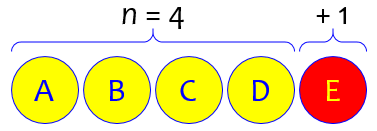Now we want to choose k + 1 of them (here, k = 2). How many of these subsets will contain only the original (yellow) items? We are choosing all 3 from among the 4: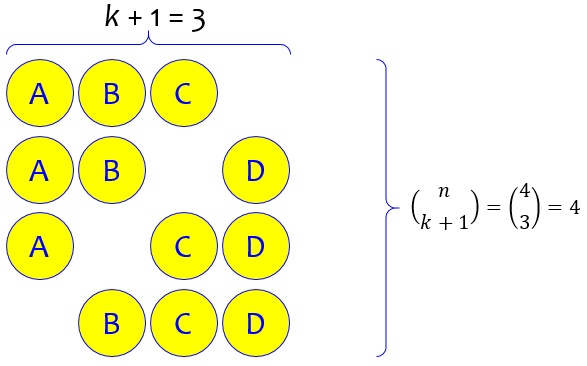How many subsets will contain the red item, along with k from the yellow ones? We are choosing only 2 from the 4, plus another: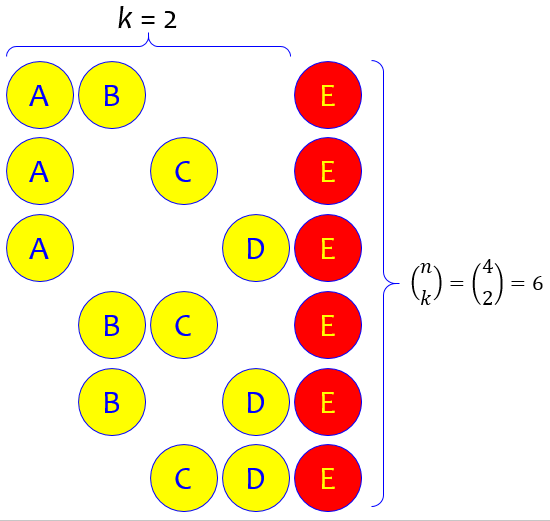How many are there in all? $${n\choose k} + {n\choose {k+1}} = {{n+1}\choose{k+1}}$$

This site uses Akismet to reduce spam. Learn how your comment data is processed.